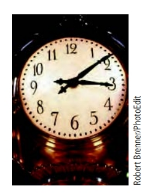Chapter 8.1, Problem 4ESMathematical Excursions (MindTap C...

4th Edition
Richard N. Aufmann + 3 others
ISBN: 9781305965584

Solutions

Chapter
SectionMathematical Excursions (MindTap C...

4th Edition
Richard N. Aufmann + 3 others
ISBN: 9781305965584
Textbook Problem

Evaluate each expression, where ⊕ and ⊖ indicate addition and subtraction, respectively, using a 12-hour clock.5 ⊕ 10

To determine

The addition 510 on a 12-hour clock.

Explanation

Given information: denote the addition on 12-hour.

Calculation:

510 denote the time 10 hours after 5 o’clock

Still sussing out bartleby?

Check out a sample textbook solution.

See a sample solution

The Solution to Your Study Problems

Bartleby provides explanations to thousands of textbook problems written by our experts, many with advanced degrees!

Get Started

Find more solutions based on key concepts